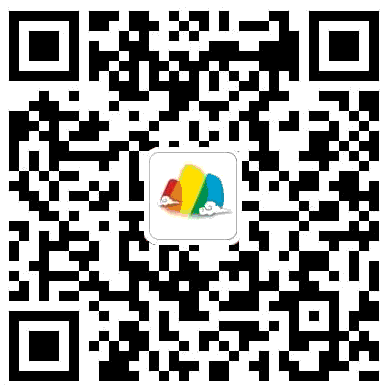# 【知识点归纳--上册（全册）】

2015-07-22 09:39:00

10506

2、通过观察等探求活动，使学生初步建立数感，初步培养学生的观察能力和口头表达。
3、使学生知道“同样多”的含义。
4、让学生在数一数、比一比、摆一摆的活动中，体验一些比较的方法比一比------同样多、多、少

目标：

1、通过直观演示和动手操作，使学生认识“上”、“下”、“前”、“后”、“左”、“右”的基本含义，初步感受它们的              相对性。

2、使学生会用“上”、“下”、“前”、“后”、“左”、“右”描述物体的相对位置。

内容：上下左右前    后:目标：

1、使学生能认、读、写5以内各，并注意书写工整。会用5以内各数表示物体的个数和事物的顺序，会区分几个和第几个。

2、使学生掌握5以内数的顺序和各数的组成。

3、使学生认识符号“＞”“＜”“＝”的含义，知道用词語（大于、小于、等于）来描述5以内数的大小。

4、使学生初步知道加减法的含义，会用自己理解的方法口算5以内的加减法。

5、能运用5以内各数表示日常生活中的一些事物，初步建立数感，运用数学来表示生活中的事物并进行交流。

内容：
认识1-5：4书写1-5：33区分几个和第几：认识“＞”“＜”“＝”符号，并用符号给5以内的数字排序：数的分合：感知0：0表示起点0表示没有口算5以内加减法：  1+1=2    1+2=3    1+3=4    1+4=5
2+1=3    2+2=4    2+3=5
3+1=4    3+2=5
4+1=5
5+0=5

5-0=5    5-1=4    5-2=3    5-3=2   5-4=1   5-5=0
4-0=4    4-1=3    4-2=2    4-3=1   4-4=0
3-0=3    3-1=2    3-2=1    3-3=0
2-0=2    2-1=1    2-2=0
1-0=1    1-1=0

目标：

1、使学生直观认识长方体、正方体、圆柱和球等立体图形与长方形、正方形、三角形和圆等平面图形，能够辨认和区别这            些图形。

2、通过、摆、画各种图形，使学生直观感受各种图形和特征。

3、培养学生初步的观察能力、动手操作能力和用数学交流和能力。

4、使学生感受数学与实际生活的联系。

5、在愉悦的氛围中激发学生的学习兴趣，培养学生合作、探究和创新意识，初步建立空间观念

内容：

认识物体和形状：圆形           正方形           圆柱             长方体

拼组立体图形：目标：1、能正确熟练的数出数量是6-10的物体的个数，并会读、写这两个数。
2、掌握10以内数的顺序，会比较它们的大小，掌握6-10的组成
3、可以解决10以内的实际问题
内容：

排大小：6----10的分合6----10的加减法：2、使学生初步认识“十位”“个位”，初步了解十进制，能够正确地迅速地读写11到20各数。
3、使学生能够熟练地口算10加几和相应的减法。

4、通过看图数数，初步培养学生有序观察、分类观察等良好的观察习惯。
5、通过摆小棒，培养学生的动手操作能力。

2、认识十位与个位。
3、熟练口算10加几和相应的减法。
4、培养学生的观察、操作能力。

认识数字11-20：。。。。。。11---20各数的顺序：10加几、十几加几与相应的减法：2、使学生结合生活经验 会看、会写钟表和电子表上整时和半时的时刻 。
3、培养学生初步的观察、分析、推理的能力。1、2、3、4、5、6、7、8、9、10、、时针、分针、秒针9＋2＝11

9＋3＝12    8+3=11

9＋4＝13    8+4=12    7+4=11

9＋5＝14    8+5=13    7+5=12    6+5=11

9＋6＝15    8+6=14    7+6=13    6+6=12    5+6=11

9＋7＝16    8+7=15    7+7=14    6+7=13    5+7=12    4+7=11

9＋8＝17    8+8=16    7+8=15    6+8=14    5+8=13    4+8=12    3+8=11

9＋9＝18    8+9=17    7+9=16    6+9=15    5+9=14    4+9=13    3+9=12    2+9=11最后编辑：Alex121 于 2015-08-07 14:33:44

发表评论
评论通过审核之后才会显示。
分类免费预约体验课